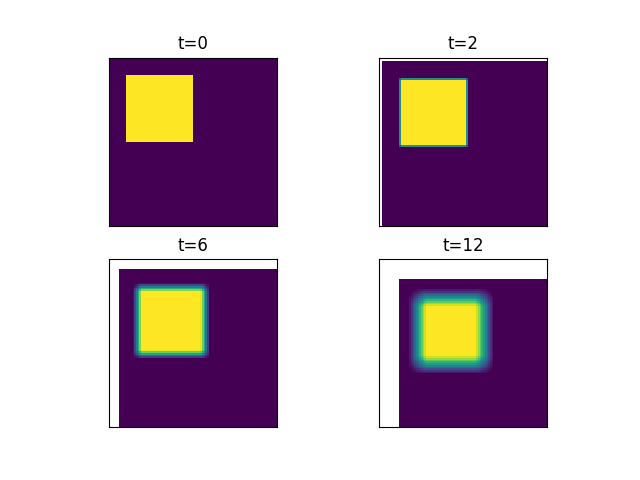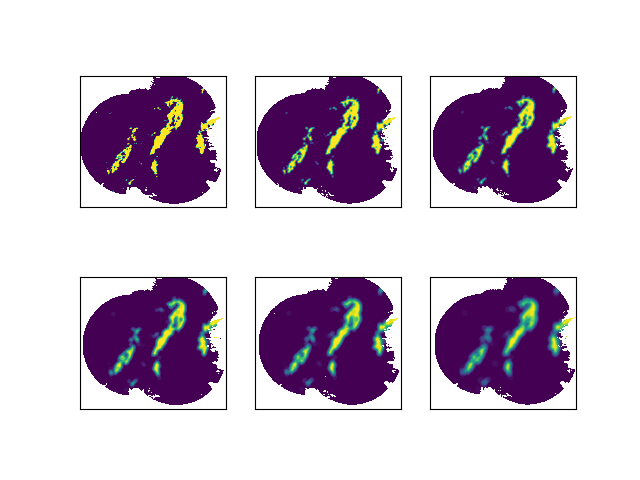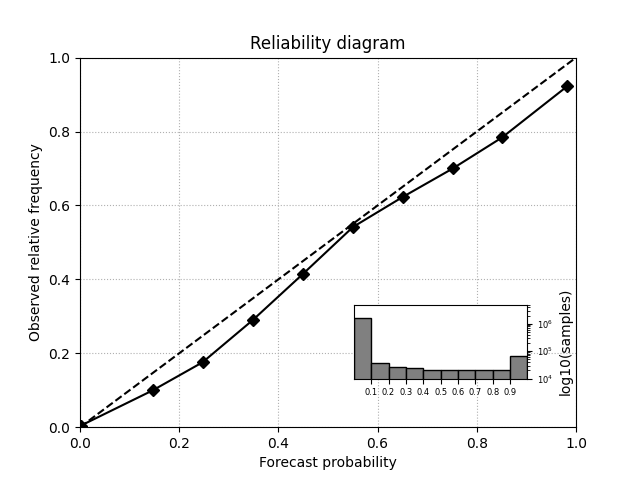# Probability forecasts#

This example script shows how to forecast the probability of exceeding an intensity threshold.

The method is based on the local Lagrangian approach described in Germann and Zawadzki (2004).

```import matplotlib.pyplot as plt
import numpy as np

from pysteps.nowcasts.lagrangian_probability import forecast
from pysteps.visualization import plot_precip_field
```

## Numerical example#

First, we use some dummy data to show the basic principle of this approach. The probability forecast is produced by sampling a spatial neighborhood that is increased as a function of lead time. As a result, the edges of the yellow square becomes more and more smooth as t increases. This represents the strong loss of predictability with lead time of any extrapolation nowcast.

```# parameters
precip = np.zeros((100, 100))
precip[10:50, 10:50] = 1
velocity = np.ones((2, *precip.shape))
timesteps = [0, 2, 6, 12]
thr = 0.5
slope = 1  # pixels / timestep

# compute probability forecast
out = forecast(precip, velocity, timesteps, thr, slope=slope)
# plot
for n, frame in enumerate(out):
plt.subplot(2, 2, n + 1)
plt.imshow(frame, interpolation="nearest", vmin=0, vmax=1)
plt.title(f"t={timesteps[n]}")
plt.xticks([])
plt.yticks([])
plt.show()
``````/home/docs/checkouts/readthedocs.org/user_builds/pysteps/envs/stable/lib/python3.8/site-packages/pysteps/nowcasts/lagrangian_probability.py:104: RuntimeWarning: invalid value encountered in divide
precip_forecast[i, ...] /= kernel_sum
```

## Real-data example#

We now apply the same method to real data. We use a slope of 1 km / minute as suggested by Germann and Zawadzki (2004), meaning that after 30 minutes, the probabilities are computed by using all pixels within a neighborhood of 30 kilometers.

```from datetime import datetime

from pysteps import io, rcparams, utils
from pysteps.verification import reldiag_init, reldiag_accum, plot_reldiag

# data source
source = rcparams.data_sources["mch"]
root = rcparams.data_sources["mch"]["root_path"]
fmt = rcparams.data_sources["mch"]["path_fmt"]
pattern = rcparams.data_sources["mch"]["fn_pattern"]
ext = rcparams.data_sources["mch"]["fn_ext"]
timestep = rcparams.data_sources["mch"]["timestep"]
importer_name = rcparams.data_sources["mch"]["importer"]
importer_kwargs = rcparams.data_sources["mch"]["importer_kwargs"]

date = datetime.strptime("201607112100", "%Y%m%d%H%M")
fns = io.find_by_date(date, root, fmt, pattern, ext, timestep, num_prev_files=2)
importer = io.get_method(importer_name, "importer")
# precip[np.isnan(precip)] = 0

# motion

# parameters
thr = 1  # mm / h
slope = 1 * timestep  # km / min

# compute probability forecast
extrap_kwargs = dict(allow_nonfinite_values=True)
fct = forecast(
precip[-1], motion, nleadtimes, thr, slope=slope, extrap_kwargs=extrap_kwargs
)

# plot
for n, frame in enumerate(fct):
plt.subplot(2, 3, n + 1)
plt.imshow(frame, interpolation="nearest", vmin=0, vmax=1)
plt.xticks([])
plt.yticks([])
plt.show()
```Let’s plot one single leadtime in more detail using the pysteps visualization functionality.

```plt.close()
# Plot the field of probabilities
plot_precip_field(
fct,
ptype="prob",
probthr=thr,
title="Exceedence probability (+ %i min)" % (nleadtimes * timestep),
)
plt.show()
``````/home/docs/checkouts/readthedocs.org/user_builds/pysteps/envs/stable/lib/python3.8/site-packages/pysteps/visualization/utils.py:427: UserWarning: cartopy package is required for the get_geogrid function but it is not installed. Ignoring geographical information.
warnings.warn(
```

## Verification#

```# verifying observations
importer = io.get_method(importer_name, "importer")
fns = io.find_by_date(
date, root, fmt, pattern, ext, timestep, num_next_files=nleadtimes
)